# Valence shell of Silicon

#### MikeJacobs

Joined Dec 7, 2019
218
So it is well known that the third valence shell of an atom can contain 18 electrons max.

However, in a silicon atom, the outermost shell is considered to be full with only 8 electrons.
Why is this?
It has something to do with energy level groups but i dont understand it.

I found this but still dont get it
Does the 3rd electron shell have a capacity for 8e- or 18e-? | Socratic

Can someone explain?

Thanks

#### wayneh

Joined Sep 9, 2010
17,172

#### MikeJacobs

Joined Dec 7, 2019
218
This makes it worse actually

In the 3rd shell you have 2 / 6 and 10

So how is 8 considered full for a silicon atom

#### Delta Prime

Joined Nov 15, 2019
1,311
Hello thereI hope this fills in some of the gaps in your research.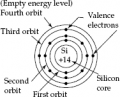Each ‘Silicon atom’ has its electrons arranged in groups of energy levels or shells as the following:
First orbit, the inner most energy level has 2 electrons (completely filled).
Second orbit has 8 electrons (completely filled).
Third orbit has the balance of 4 electrons (partially filled).
Energy levels starting from the fourth level are empty energy levels.
This last partially filled shell (third orbit) is called valence shell.
The 4 electrons in the third orbit (shell) are known as valence electrons.
Valence electrons are responsible for the chemical and electrical properties of the material.
Electrons extracted from valence shell and not subject to force of attraction of nucleus on them are called free electrons.
basis to understand the concept of covalent bond formation etc. Silicon semiconductor, as a ‘Tetravalent’ material, has ‘four valence electrons’. The force of attraction between the nucleus (core) and the electron inside the atom is given by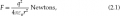where electronic charge q is in coulombs, r is the separation distance between electrons and nucleus (in an atom) in metres, the force F is in Newtons, ɛ0 is the permittivity of free space in farads/metre, and permittivity of the free space ɛ0 = 8.849 × 10–12 farads/meter.
This force of attraction F between the nucleus and the electron is counter balanced by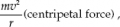where m is the electronic mass, m = 9.109 × 10–31 kg, v is the speed of the electron in the orbit, acceleleration a = v2/r and is directed towards the nuclei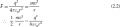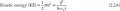The potential energy PE of the electron at a distance ‘r’ from the nucleus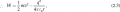•MikeJacobs

#### MikeJacobs

Joined Dec 7, 2019
218
Hello thereI hope this fills in some of the gaps in your research.

View attachment 231988

Each ‘Silicon atom’ has its electrons arranged in groups of energy levels or shells as the following:
First orbit, the inner most energy level has 2 electrons (completely filled).
Second orbit has 8 electrons (completely filled).
Third orbit has the balance of 4 electrons (partially filled).
Energy levels starting from the fourth level are empty energy levels.
This last partially filled shell (third orbit) is called valence shell.
The 4 electrons in the third orbit (shell) are known as valence electrons.
Valence electrons are responsible for the chemical and electrical properties of the material.
Electrons extracted from valence shell and not subject to force of attraction of nucleus on them are called free electrons.
basis to understand the concept of covalent bond formation etc. Silicon semiconductor, as a ‘Tetravalent’ material, has ‘four valence electrons’. The force of attraction between the nucleus (core) and the electron inside the atom is given by
View attachment 231979
where electronic charge q is in coulombs, r is the separation distance between electrons and nucleus (in an atom) in metres, the force F is in Newtons, ɛ0 is the permittivity of free space in farads/metre, and permittivity of the free space ɛ0 = 8.849 × 10–12 farads/meter.
This force of attraction F between the nucleus and the electron is counter balanced by
View attachment 231982
where m is the electronic mass, m = 9.109 × 10–31 kg, v is the speed of the electron in the orbit, acceleleration a = v2/r and is directed towards the nuclei
View attachment 231984
View attachment 231985

The potential energy PE of the electron at a distance ‘r’ from the nucleus
View attachment 231986
You are awesome!

•Delta Prime